# Go Math Grade 3 Answer Key Chapter 3 Understand Multiplication Assessment Test

Go Math Grade 3 Answer Key Chapter 3 contains all the topics of chapter 3 which helps to test the student’s knowledge. Through this assessment test, students can check their knowledge. This assessment test is also helpful for the teachers to know how much a student understood the topics.

This chapter can improve student’s math skills, by referring to the Go Math Grade 3 Answer Key Chapter 3 Understand Multiplication Assessment Test, and with the help of this Go Math Grade 3 Assessment Test Answer Key, students can score good marks in the examination.

Chapter 3: Understand Multiplication Assessment Test

### Test – Page 1 – Page No. 31

Question 1.
There are 4 flower beds in Max’s yard. Three rosebushes grow in each flower bed. How many rosebushes are there? Draw circles to model the problem and explain
how to solve it.
_______ rosebushes

Explanation: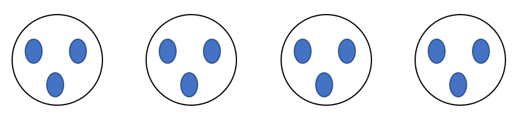In the above figure, each big circle represents a flower bed and three small circles represent rosebushes. By using this model students can count the number of circles or add 3 + 3+ 3+ 3 = 12 or multiply 4 (flower beds) × 3 (rosebushes) = 12 rosebushes

Question 2.
Greta put 6 coins into each of 3 stacks. She wrote this number sentence to represent the total number of coins.
3 × 6 = 18
What is a related number sentence that also represents the total number of coins she has?
Options:
a. 6 × 3 = ■
b. 6 + 3 = ■
c. 3 + 3 + 3 = ■
d 6 × 6 = ■

Explanation: For the written number sentence by 3 × 6 = 18 Greeta, only option a. 6 × 3 = 18 matches. The remaining options don’t give an answer as option a.

Question 3.
Cecile went fishing for three days at a lake. The first jump on the number line shows how many fish she caught the first day. She caught the same number of fish the next two days.Write the multiplication sentence that the number line shows.
_____ × _____ = _____

Answer: 3 × 3 = 9

Explanation:
Cecile made 3 jumps, for every jump she caught 3 fishes
Therefore  3 × 3 = 9.

### Test – Page 2 – Page No. 32

Question 4.
Ben drew an array to show the number of video games he has.
Write a multiplication sentence for the array._____ video games

Answer: 8 × 2 = 16.

Explanation:
In the given array each row contains 8 video games, total it has 2 rows
Therefore multiplication sentence for the array = 8 × 2 = 16.

Question 5.
Julissa makes 4 bracelets. She uses 9 charms on each bracelet.
For numbers 5a–5d, tell if the number sentence could be used to find the number of charms Julissa uses.
a. 4 + 9 = ■
i. yes
ii. no

Explanation: He cannot use  4 + 9 in this sentence as it gives result = 13 which not correct.

Question 5.
b. 3 + 3 + 3 + 3 = ■
i. yes
ii. no

Explanation: He cannot use 3 + 3 + 3 + 3 this sentence as it gives result = 13 which not correct.

Question 5.
c. 9 + 9 + 9 + 9 = ■
i. yes
ii. no

Explanation: He can use 9 + 9 + 9 + 9 = 36, which gives no of charms used on 4 bracelets.

Question 5.
d. 4 × 9 = ■
i. yes
ii. no

Explanation: He can use 4 × 9 = 36, which gives no of charms used on 4 bracelets.

Question 6.
Edith sorts buttons into 4 groups for her art project. Each group contains 6 buttons. How many buttons does Edith sort? Make a bar model to solve the problem.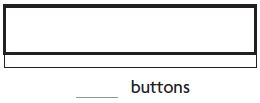_____ buttons

Explanation: Given that Edith sorts buttons into 4 groups, where each group contains 6 buttons.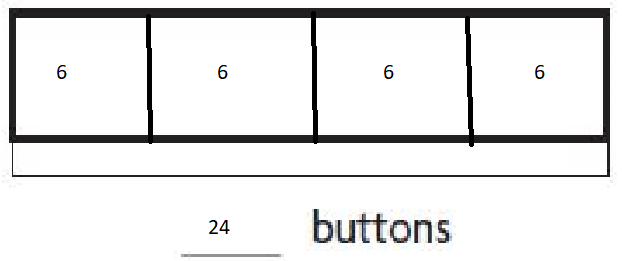From the given info, we drew the above diagram, from the above model total no of buttons = 6+6+6+6 = 24 buttons

Question 7.
Select the number sentences that show the Commutative Property of Multiplication. Mark all that apply.
Options:
a. 5 × 2 = 5 + 5
b. 6 × 0 = 6
c. 7 × 5 = 5 × 7
d. 8 × 1 = 1 × 8
e. 9 × 1 = 9

Explanation:
Commutative Property of Multiplication means a × b = b × a.
Therefore options b and c,  7 × 5 = 5 × 7 and 8 × 1 = 1 × 8 shows the property.

### Test – Page 3 – Page No. 33

Question 8.
There are 5 tables in the library. Four students are sitting at each table. How many students are sitting in the library?
_____ students

Answer: 5 × 4 = 20 students

Explanation:
Given that there 5 tables in the library, 4 students are sitting on each table
Therefore total no of students = 5 × 4 = 20 students.

Question 9.
Keisha needs 3 equal lengths of rope for a Field Day activity. The jump on the number line shows the length of one rope in yards. How many yards of rope does Keisha need?_____ yards

Explanation:
Given that there are 3 equal length rope
From the number line length of the one rope = 5 yards
Since he is having 3 ropes, the total length of all the 3 ropes = 5 × 3 = 15 yards.

Question 10.
Anna’s mom makes 3 sandwiches every school day. Each sandwich gets 3 slices of cheese. How many slices of cheese will Anna’s mom need for all the sandwiches she makes on 2 school days?
_____ slices of cheese

Explanation:   No of Sandwiches Anna’s mom make = 3
Each sandwich having 3 slices of cheese, no of cheese in 3 sandwiches = 3 × 3 = 9
Therefore to make 3 sandwiches for 2 days, the number of cheese slices needed = (3 ×3) × 2 = 18.

Question 11.
Angelo stacked 30 cans of soup collected during a food drive.Select other ways Angelo could arrange the same number of cans. Mark all that apply.
Options:
a. 1 row of 30
b. 5 rows of 6
c. 6 rows of 6
d. 8 rows of 4
e. 10 rows of 3

Explanation: Total no of soup cans = 30, therefore only by arranging as given in options a, b, e, gives us 30 cans.

### Test – Page 4 – Page No. 34

Question 12.
Choose the number that makes the sentence true.
The product of any number andis zero.
_____

Answer: Product of any number and zero is zero

Explanation:

The product of any number and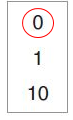is zero.

Question 13.
Ellen made this array to show that 2 × 9 = 18.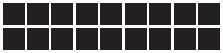Part A
Ellen says that 9 × 2 = 18. Is Ellen correct? Draw an array to explain your answer.
i. yes
ii. no

Explanation: Yes, Ellen is correct. 9 × 2 = 18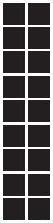Question 13.
Part B
________

Explanation: The given array shows 2 × 9 = 18 and 9 × 2 = 18, which is a Commutative Property of Multiplication, so Ellen is correct.

Question 14.
Abdul has a collection of stamps. He puts the stamps in 2 equal groups. There are 7 stamps in each group. How many stamps does Abdul have? Use the number line to show your work._____ stamps

Explanation:
Given that Abdul has 2 equal groups of stamps
Each group contains 7 stamps in it, so total stamps in both groups = 7 × 2 = 14 stamps.

### Test – Page 5 – Page No. 35

Question 15.
Hudson and Asher each collect comic books.
Part A
Hudson sorts his comic books into 3 piles. Each group has 7 comic books. How many comic books does he have?
_____ comic books

Explanation:
Given that Hudson sorted his books into 3 piles
Each group has 7 comic books
Total no of comic books in 3 piles = 3 × 7 = 21.

Question 15.
Part B
Asher sorts his comic books into 4 piles. Each pile has 2 comic books in it. Write a multiplication sentence to show how many comic books Asher has.
Then find how many comic books Hudson and Asher have.

Answer: 2 × 4 = 8; 21 + 8 = 29 comic books

Explanation:
Given that Asher sorted his comic books into 4 piles
Each group has 2 comic books
Total no of comic books in 3 piles = 2 × 4 = 8
Total no of comic books both Hudson and Asher have = 21 + 8 = 29.

Question 16.
Aiden sees 4 lifeguard towers at the beach. Each tower has 1 lifeguard. Write a multiplication sentence to show the number of lifeguards Aiden sees.
_____ × _____ = _____ lifeguard towers

Explanation: 4 × 1 = 4 lifeguards.

Question 17.
Jorge spends 7 minutes completing each of 4 puzzles. He can use 7 × 4 to find the total amount of time he spends on the puzzles.
For numbers 17a–17d, choose Yes or No to show which expressions are equal to 7 × 4 .
a. 7 + 4
i. yes
ii. no

Explanation: No, 7 × 4 = 28, where in 7 + 4 = 11.

Question 17.
b. 7 + 7 + 7 + 7
i. yes
ii. no

Explanation: Yes, 7 × 4 = 28, where in 7 + 7 + 7 + 7 = 28.

Question 17.
c. 4 + 4 + 4 + 4 + 4 + 4 + 4
i. yes
ii. no

Explanation: Yes, 7 × 4 = 28, where in 4 + 4 + 4 + 4 + 4 + 4 + 4 = 28.

Question 17.
d. 7 + 7 + 7 + 7 + 7 + 7 + 7
i. yes
ii. no

Explanation: No, 7 × 4 = 28, where in 7 + 7 + 7 + 7 + 7 + 7 + 7 = 49.

### Test – Page 6 – Page No. 36

Question 18.
Maya buys 3 bags of dried pears. Each bag contains 6 dried pears.
Select the number sentences that show all the dried pears Maya buys. Mark all that apply.
Options:
a. 3 + 3 + 3 = 9
b. 3 + 3 + 3 + 3 + 3 + 3 = 18
c. 6 + 3 = 9
d. 6 + 6 + 6 = 18
e. 3 × 6 = 18
f. 9 + 9 = 18

Explanation:
Given that Maya buys 3 bags of dried pears
Each bag contains 6 dried pears = 3 × 6 = 18
Therefore options b, d, and e match the dried pears Maya buys.

Question 19.
Javier is making 6 smoothies. He puts 4 strawberries and 1 banana in each smoothie.
Part A
Write the total number of strawberries and bananas he uses. Write a multiplication sentence for each
______ strawberries            ______ bananas

Answer: 6 × 4 = 24 strawberries, 6 × 1 = 6 bananas.

Explanation:
Given that Javier is making 6 smoothies, uses 4 strawberries and 1 banana in each smoothie
Therefore to make 6 smoothies no of strawberries needed = 6 × 4 = 24 strawberries,
And the number of bananas needed to make 6 smoothies = 6 × 1 = 6 bananas.

Question 19.
Part B
After making 6 smoothies, Javier has 9 strawberries and 4 bananas left. What is the greatest number of smoothies he can make with that fruit if he uses the same recipe for all the smoothies? Draw models and use them to explain.

Answer: At most, he can make 2 more smoothies.

Explanation:He can make 4 smoothies with 1 banana each, but only 2 smoothies with 4 strawberries each, so the greatest number of smoothies he can make is 2. There will be 2 bananas and 1 strawberry left.

Conclusion:

This assessment test helps students to check their math skills. Go Math Grade 3 Chapter 3 questions are explained in detail that students can understand easily.

Scroll to Top